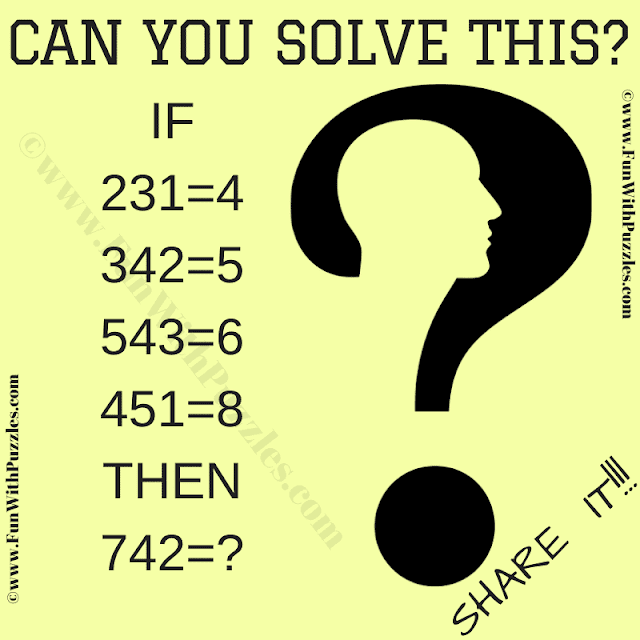This is a genius cracking code puzzle that will twist your brain. In this tough math logic puzzle, you are shown some number equations. There is one hidden mathematical or logical code that makes these number equations correct. Your challenge in this puzzle is to crack this hidden code. Once you were able to decipher the hidden code, find the value of the missing number in the last equation.
This is a tough logical number puzzle for genius brains. I will be publishing the answer to this puzzle later once there are few comments from the readers. If you can solve this puzzle, please write down your answer along with the logical reasoning in the comments box.Can you solve this Genius Cracking Code Puzzle?

The answer to this "Number Puzzle", can be viewed by clicking on the answer button.

If the given number equations are ABC=D then the hidden logical code in this genius puzzle is as below
ABC can be divided into two different numbers, one number is represented by AB and another number by C. Let's say that EF is the digits obtained from the difference of these two numbers then D = E+F

Unknown said...

9

Unknown said...

If the number is considered as 'ABC', the equation is
A+(B-C). So,
231 = 2+(3-1) = 4 and so on.
742 = 7 + (4-2) 9

Rajesh Kumar said...

Yes, your answer and logical reasoning to solve this puzzle is correct.

Unknown said...

I've come up with another solution...
here it is
231=4(13+2=15)(5-1=4)
342=5(24+3=27)(7-2=5)
543=6(34+5=39)(9-3=6)
451=8(15+4=19)(9-1=8)
so
742=2(24+7=31)(3-1=2)

Rajesh Kumar said...

Your answer is logically correct. Thanks to your out of box thinking to come up with the different logic and answer to this genius cracking code puzzle :).

Unknown said...

I really feel nervous that i didn't spot the obvious solution from the beginning

Rajesh Kumar said...

This is because of the Genius word added to the post title. If the title would have been the "Simple Cracking Code Puzzle", then our mind will try to find the simple solution. However because the title contains the word "Genius", our mind will try to find a complex solution.

Unknown said...

In my opinion,the simplier you think,the smarter you are.Thanks for your comments though.

Unknown said...

The answer could also be (-2).If you look at the way I substracted the numbers,you will see that in the last one I did it in reverse order.

Fun With Puzzles said...

Yes, you are right. One can take the absolute value or else the answer could be -2.